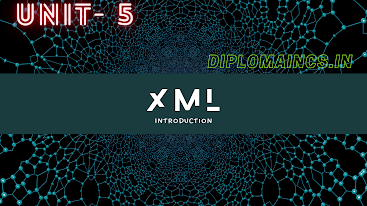## 2/28/20

### Computer programming through C previous year questions 2019 odd

Sub Code: - 1600302

2019(ODD)
Time : 3Hrs
Semester  III(New)
CPTC
Full Marks : 70
Pass marks : 28

Group A

Choose the most suitable answer from the following options: -   (1*20=20)

(i) Which of the following special symbol allowed in a variable name?

(a) * (Asterisk)
(b) | (Pipeline)
(c) – (Hyphen)
(d) _ (Underscore)

(ii) Which of the following is not a keyword in C language?

(a) Getchar
(b) Int
(c) Void
(d) Volatile

(iii) Which header file is designed for basis mathematical operations?

(a) Stdio.h
(b) Dos.h
(c) Math.h
(d) Conio.h

(iv) What is the symbol used for conditional operator?

(a)==
(b) /
(c) ?=
(d)  ?:

(v) What is the extension file by default for C program?

(a) “.doc”
(b) “.ppt”
(c) “.c”
(d) “.exe”

(vi) The structure combines variables of _______ data type.

(a) Similar
(b) Variable
(c) Dissimilar
(d) None of these

(vii) The ____ statement causes the loop to be terminated.

(a) Continue
(b) Default
(c) Break
(d) Exit

(viii) Which of the following statement is false in C

(a) Variables name do not contain a blank space.
(b) Keywords can be used as variables names
(c) Capital letters can be used in variable name
(d) Variable names can contain a digit.

(ix) Which of the following is the correct way of declaring a float pointer:

(a) float * ptr;
(b) * float ptr;
(c) float ptr;
(d) None of the above

(x)  Which committee standardized c programming language?

(a) ISO
(b) TRAI
(c) ANSI
(d) W3C

(xi) Which of the following cannot be checked in a switch case statement?

(a) Float
(b) Integer
(c) Character
(d) None of the above

(xii) Which one of the following is a bitwise operator?

(a) ==
(b) =
(c) <
(d) <<

(xiii) Which one is the special operator?

(a) <<
(b) ++
(c) ?:
(d) size of()

(xiv) Which of the following is example of call by value?

(a) num (*a, *b)
(b) num (a,b)
(c) num (&a, &b)
(d) None of the above

(xv) Which is syntax of for loop?

(a) for (initialization; condition; increment/decrement)
(b) for (increment; expression; condition)
(c) for (condition; expression; statement)
(d) for (expression; condition; statement)

(xvi) How many elements can be store in the array A

(a) 36
(b) 37
(c) 35
(d) 34

(xvii) How many of bytes in memory required for single character in computer?

(a) 8 bytes
(b) 2 bytes
(c) 4 bytes
(d) 1 bytes

(xviii) Which of the following is correct precedence order from higher to lower?

(a) (),<<,*,+
(b) (),*,<<,+
(c) (),*,+,<<
(d)  *,<<,(),+

(xix) What is the value range of data type unsigned short int

(a) -128 to 127
(b) 0 to 255
(c) 0 to 65535
(d) 0 to 128

(xx) P++ is called______ operator

(a) Decrement
(b) Post decrement
(c) Post increment
(d) Pre increment

Group:-"B"

Answer all Five Questions: -                         (5*4=20)

2. Define an algorithm. Write an algorithm t0 convert temperature Fahrenheit to Celsius.
OR
Explain different types of programming languages.

3.  Write a ‘C’ program to find the biggest of given two numbers using conditional operator.
OR
What is an operator? Describe different types of operator used in ‘C’ programming.

4. What is structure in C? How structure is declared?
OR
What is pointer? How a pointer variable is declared and initialize?

5. Define the function with suitable examples.
OR
What is call by value and call by reference? Explain with suitable example.

6. Write a ‘C’ program to find the biggest number in a given array.
OR
Write a ‘C’ program to input 20 students name and print them.

Group:- "C"

Answer all Five Questions: -                         (5*6=30)

7. Write a ‘C’ program to find the value of Y using
y (x,n)=1+x, when n=1
=1 + x/n, when n =2
=1 + x to the power n, when n=3
=1+ n to the power x, when n>3 or n<1 o:p="">
(By the help of nested if)
OR
Write a ‘C’ program to print the following outputs using for loops
5 5 5 5 5
4 4 4 4
3 3 3
2 2
1

8. Define the data types of C-language. How many data types are available in C language?
OR
If ages of Ram, Shyam, and Ajay are input through the keyword. Write a program to determine the youngest of the three.

9. If lengths of the three sides of a triangle are input through keyboard, write a program to find the area of the triangle.
OR
What is an array? Write an algorithm to search an element from an array.

10. Define the switch statement with suitable example.
OR
What is loop? Define for loop with syntax and example.

11. Write a ‘C’ program to find square root of quadratic equation.
OR
What is token? How many types of token are used in ‘C’ language? Explain in detail.